Document revision date: 15 July 2002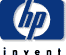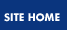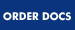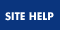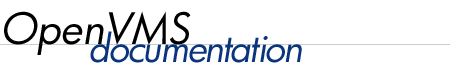## OpenVMS User's Manual

 Previous Contents Index

### 12.8.3 Logical Operation Results

The following tables demonstrate the results of logical operations on a bit-by-bit basis and a number-by-number basis. In logical operations, a character string beginning with an uppercase or lowercase T or Y is treated as the number 1; a character string beginning with any other character is treated as the number 0. In logical operations, odd numbers are true and even numbers and zero are false.

Given That:     The Results Are:
Bit A Bit B   .NOT. A A .AND. B A .OR. B
1 1   0 1 1
1 0   0 0 1
0 1   1 0 1
0 0   1 0 0
Given That:     The Results Are:
Number A Number B   .NOT. A A .AND. B A .OR. B
odd odd   even odd odd
odd even   even even odd
even odd   odd even odd
even even   odd even even

### 12.8.4 Using Values Returned by Lexical Functions

Typically used in command procedures, lexical functions retrieve information from the system, including information about system processes, batch and print queues, and user processes. You can also use lexical functions to manipulate character strings and translate logical names. When you assign a lexical function to a symbol, the symbol is equated to the information returned by the lexical function (for example, a number or character string). At DCL level, you can then display that information with the DCL command SHOW SYMBOL. In a command procedure, the information stored in the symbol can be used later in the procedure. See Chapter 15 for additional information on lexical functions.

To use a lexical function, specify the name of the lexical function (which always begins with F\$) and its argument list. Use the following format:
 F\$function-name(args[,...])

The argument list follows the function name with any number of intervening spaces and tabs.

When you use a lexical function, observe the following rules:

• Enclose the argument list in parentheses.
• Within the list, specify arguments in exact order and separate them with commas; even if you omit an optional argument, do not omit the comma.
• If no arguments are required, type an empty set of parentheses.
• Follow the rules for writing expressions: enclose character strings in quotation marks; do not enclose integers, symbols, and lexical functions in quotation marks.

Use lexical functions the same way you would use character strings, integers, and symbols. When you use a lexical function in an expression, DCL automatically evaluates the function and replaces the function with its return value.

In the following example, the F\$LENGTH function returns the length of the value specified as BUMBLEBEE as its argument. DCL automatically determines the return value (9) and uses this value to evaluate the expression.
Therefore, the result of the expression (9 + 1) is 10 and this value is assigned to the symbol SUM:

 ```\$ SUM = F\$LENGTH("BUMBLEBEE") + 1 \$ SHOW SYMBOL SUM SUM = 10 Hex = 0000000A Octal = 00000000012 ```

Note that each lexical function returns information as either an integer or a character string. In addition, you must specify the arguments for a lexical function as either integer or character string expressions.

For example, the F\$LENGTH function requires an argument that is a character string expression and it returns a value that is an integer. In a previous example, the argument "BUMBLEBEE" is a character string expression and the return value (9) is an integer.

You can use a lexical function in any position that you can use a symbol. In positions where symbol substitution must be forced by enclosing the symbol in apostrophes (see Section 12.12), lexical function evaluation must be forced by placing the lexical function within apostrophes. Lexical functions can also be used as argument values in other lexical functions.

The following examples show different ways you can specify the argument for the F\$LENGTH function. In each example, the argument is a character string expression.

• The following example shows a symbol that is used as an argument:

 ```\$ BUG = "BUMBLEBEE" \$ LEN = F\$LENGTH(BUG) \$ SHOW SYMBOL LEN LEN = 9 Hex = 00000009 Octal = 00000000011 ```

When you use the symbol BUG as an argument, do not place quotation marks around it. The lexical function automatically substitutes the value "BUMBLEBEE" for BUG, determines the length, and returns the value 9.

• The following example shows an argument that contains both a symbol and a character string:

 ```\$ BUG = "BUMBLEBEE" \$ LEN = F\$LENGTH(BUG) \$ SHOW SYMBOL LEN LEN = 9 Hex = 00000009 Octal = 00000000011 \$ LEN = F\$LENGTH(BUG + "S") \$ SHOW SYMBOL LEN LEN = 10 Hex = 0000000A Octal = 00000000012 ```

The symbol BUG is not enclosed in quotation marks but the string "S" is. The argument must be evaluated before the F\$LENGTH function can determine the length. The value represented by the symbol BUG ("BUMBLEBEE") is concatenated with the string "S"; the result is "BUMBLEBEES". The F\$LENGTH function determines the length of the string "BUMBLEBEES" and returns the value 10.

• The following example uses another lexical function as the argument for the F\$LENGTH function. The F\$DIRECTORY function returns the name of your current default directory, including the square brackets. In the following example, the current default directory is [SALMON].

 ```\$ LEN = F\$LENGTH(F\$DIRECTORY()) \$ SHOW SYMBOL LEN LEN = 8 Hex = 00000008 Octal = 00000000010 ```

Do not place quotation marks around the F\$DIRECTORY function when it is used as an argument; the function is automatically evaluated. The result of the F\$DIRECTORY function must be returned before the F\$LENGTH function can determine the length. Then, the F\$LENGTH function determines the length of your default directory, including the square brackets.

### 12.8.5 Order of Operations

An expression can contain any number of operations and comparisons. The following table lists the operators in the order in which they are evaluated if there are two or more operators in an expression. The operators are listed from highest to lowest precedence; that is, operators at the top of the table are performed before operators at the bottom.
Precedence Operation
7 Unary plus (+) and minus (--)
6 Multiplication (*) and division (/)
5 Addition (concatenation) and subtraction (reduction)
4 All numeric and character comparisons
3 Logical .NOT. operations
2 Logical .AND. operations
1 Logical .OR. operations

If an expression contains operators that have the same order of precedence, the operations are performed from left to right. You can override the normal order of precedence (the order in which operation and comparison would be evaluated) by placing operations to be performed first in parentheses. Parentheses can also be nested.

In the following example, the parentheses force the addition to be performed before the multiplication. Without the parentheses, the multiplication is performed first and the result is 26:

 ```\$ RESULT = 4 * (6 + 2) \$ SHOW SYMBOL RESULT RESULT = 32 Hex = 00000020 Octal = 00000000040 ```

### 12.8.6 Evaluating Data Types

The result of DCL's evaluation of a symbol is either a character string or an integer value. The data type (character or integer) of a symbol is determined by the data type of the value currently assigned. The data type is not permanent: if the value changes data type, the symbol changes data type.

An expression has either an integer or a string value, depending on the types of values and the operators used.

In the following example, the local symbol NUM is first assigned a character value and then converted to an integer value when assigned an integer expression:

 ```\$ NUM = "ABC" \$ NUM = 2 + 5 ```

The following table summarizes how DCL evaluates expressions. The first column lists the different values and operators that an expression might contain. The second column tells, for each case, what the entire expression is equated to. Within the table any value stands for a string or an integer.
Expression Resulting
Value Type
Integer value Integer
String value String
Integer lexical function Integer
String lexical function String
Integer symbol Integer
String symbol String
+, - , or .NOT. any value Integer
Any value .AND. or .OR. any value Integer
String + or - string String
Integer + or - any value Integer
Any value + or - integer Integer
Any value * or / any value Integer
Any value (string comparison) any value Integer
Any value (numeric comparison) any value Integer

## 12.9 Converting Value Types in Expressions

All the operands in an expression must be of the same value data type before DCL can evaluate the expression. Values either have string or integer data types. String data includes character strings, symbols with string values, and lexical functions that return string values. Integer data includes integers, symbols with integer values, and lexical functions that return integer values. When an expression contains both number and string operands, DCL either converts all strings to integers or all integers to strings.

In general, if you use both string and integer values, the string values are converted to integers. The only exception is when DCL performs string comparisons. In these comparisons, integers are converted to strings.

In addition, the following lexical functions let you determine or change the value of an expression:

• F\$TYPE --- Determines the current value type of a symbol
• F\$INTEGER --- Converts a string expression to an integer value
• F\$STRING --- Converts an integer expression to a string value

### 12.9.1 Converting Strings to Integers

Character strings are converted to integers in the following ways:

• Strings containing numbers are converted to their integer values. For example, the string "45" is converted to the integer 45.
• If a character string begins with T, t, Y, or y, it is converted to the integer 1.
• If a string begins with any other letter, it is converted to the integer 0.

The following table shows examples of strings converted to integer values:
String Resulting Integer
"123" 123
"12XY" 0 (False)
"Test" 1 (True)
"hello" 0 (False)

### 12.9.2 Converting Integers to Strings

When integers are converted to character strings, the resulting string contains numbers that correspond to the integer value. The following table shows how integers are converted to string values:
Integer Resulting String
123 "123"
1 "1"
0 "0"

## 12.10 Understanding Symbol Tables

Symbols are stored in local or global symbol tables, which are maintained by the operating system.

### 12.10.1 Local Symbol Tables

DCL maintains a local symbol table for your main process and for every command level that you create when you execute a command procedure, use the CALL command, or submit a batch job. When you create a local symbol, DCL places the symbol in the local symbol table for the current command level. As long as the command level is active, DCL maintains the local symbol table for that command level; when a command level is no longer active, its local symbol table (and all the symbols it contains) is deleted. See Chapter 16 for more information about processes, command procedures, and batch jobs.

In addition to the local symbols you create, a local symbol table contains eight symbols that are maintained by DCL. These symbols, named P1, P2, and so on through P8, are used for passing parameters to a command procedure. Parameters passed to a command procedure are regarded as character strings. Otherwise, P1 to P8 are defined as null character strings (""). They are stored in the local symbol table.

### 12.10.2 Global Symbol Tables

DCL maintains only one global symbol table for the duration of a process and places all global symbols in that table. In addition to the global symbols you create, the global symbol table contains the reserved global symbols. These global symbols give you status information on your programs and command procedures as well as on system commands and utilities.

\$STATUS is the condition code returned by the most recently executed command. The symbol \$STATUS conforms to the format of an OpenVMS operating system message code. Applications programs can set the value of the global symbol \$STATUS by including a parameter value to the EXIT command. The system uses the value of \$STATUS to determine which message, if any, to display and whether to continue execution at the next higher command level. The value of the lowest three bits in \$STATUS is placed in the global symbol \$SEVERITY.

\$SEVERITY is the severity level of the condition code returned by the most recently executed command. The symbol \$SEVERITY, which is equal to the lowest three bits of \$STATUS, can have the following values:
 0 Warning 1 Success 2 Error 3 Information 4 Severe (fatal) error

\$RESTART has the value TRUE if a batch job was restarted after it was interrupted by a system failure. Otherwise, \$RESTART has the value FALSE.

### 12.10.3 Symbol Table Search Order

When the command interpreter determines the value of a symbol, it searches symbol tables in the following order:

1. The local symbol table for the current command level
2. Local symbol tables for each previous command level, searching backwards from the current level
3. The global symbol table

## 12.11 Masking the Value of Symbols

The following sections describe how to mask the value symbols.

### 12.11.1 SET SYMBOL Command

By default, all symbols (both global and local) defined in an outer command procedure level are accessible to inner procedure levels. However, you can isolate the local or global symbols in a command procedure from the symbols defined in other command procedures by using the SET SYMBOL command. The SET SYMBOL command masks the values of local and global symbols without deleting them. Thus, if a command procedure executes another command procedure, you can use the same symbol names in both procedures if you specify the SET SYMBOL command in the second procedure.

The SET SYMBOL command also controls whether DCL attempts to translate the verb string (the first word on the command line) as a symbol before processing the line. The default behavior is that the translation is attempted. The advantage to changing this behavior is that a command procedure is not affected by outer-procedure-level environments when it invokes a command.

### 12.11.2 Symbol Scoping State

The symbol scope is different for local and global symbols. When you exit a procedure level to return to a previous procedure, the symbol scoping context from the previous level is restored for both local and global symbols.

To display the current, general symbol scoping state, use the lexical function F\$ENVIRONMENT("SYMBOL_SCOPE"). To display the current verb scoping state, use the lexical function F\$ENVIRONMENT("VERB_SCOPE").

Local symbols are procedure-level dependent. If you define a local symbol in an outer procedure level, the symbol can be read (but not written to) at any inner procedure level. If you assign a value to a symbol that is local to an outer procedure level, a new symbol is created at the current procedure level. However, the symbol in the outer procedure level is not modified.

The SET SYMBOL/SCOPE=NOLOCAL command causes all local symbols defined at an outer procedure level to be inaccessible to the current procedure level and any inner levels. For example, if you specify SET SYMBOL/SCOPE=NOLOCAL at procedure levels 2 and 4:

• Procedure level 2 can read and write to level 2 local symbols only.
• Procedure level 3 can read (but not write to) level 2 local symbols. Level 3 can also read and write to level 3 local symbols.
• Procedure level 4 can read and write to level 4 local symbols only.

Global symbols are procedure-level independent. The current global symbol scoping context is applied subsequently to all procedure levels.

The /SCOPE=NOGLOBAL qualifier causes all global symbols to become inaccessible for all subsequent commands until either the /SCOPE=GLOBAL qualifier is specified or the procedure exits to a previous level at which global symbols were accessible. In addition, specifying the /SCOPE=NOGLOBAL qualifier prevents you from creating any new global symbols until the /SCOPE=GLOBAL qualifier is specified.

 Previous Next Contents Index